Next: Emf and Internal Resistance Up: Electric Current Previous: Ohm's Law

## Resistance and Resistivity

Let us attempt to find a microscopic explanation for electrical resistance and Ohm's law. Now, electric current in metals, and most other conductors found in conventional electric circuits (good or bad), is carried by free electrons. Consider a uniform wire of cross-sectional areaand length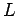made of some conducting material. Suppose that the potential difference between the two ends of the wire is. The longitudinal electric field inside the wire is therefore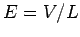. Consider a free electron of chargeand massinside the wire. The electric field in the wire exerts a force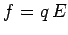on the electron, causing it to accelerate with an acceleration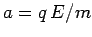along the direction of the wire. However, the electron does not accelerate for ever. Eventually, it crashes into one of the atoms in the wire. Since atoms are far more massive than electrons, the electron loses all forward momentum every time it hits an atom (just as we would lose all forward momentum if we ran into a brick wall). Suppose that the average time interval between collisions is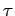. Of course, this characteristic time interval depends on the size and number density of the atoms in the wire. Immediately after the electron hits an atom (at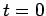, say) its forward velocityis zero. The electron is then accelerated by the electric field, so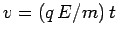. The final velocity of the electron is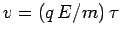, and its average velocity is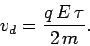(128)

In fact, on average, the electron acts as though it drifts along the wire with the constant velocity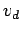. This velocity is therefore called the drift velocity.

Suppose that there arefree electrons per unit volume in the wire. All of these electrons effectively drift along the wire with the drift velocity. Thus, the total charge which passes any particular point on the wire in a time interval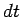is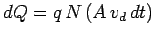. This follows because all free electrons contained in a tube of length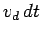and cross-sectional areapass the point in question in the time interval. The electric currentflowing in the wire is given by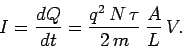(129)

This equation can be rearranged to give Ohm's law,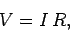(130)

where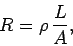(131)

and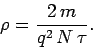(132)

Thus, we can indeed account for Ohm's law on a microscopic level. According to Eq. (131), the resistance of a wire is proportional to its length, and inversely proportional to its cross-sectional area. The constant of proportionality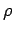is called the resistivity of the material making up the wire. The units of resistivity are ohm-meters (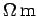). Table 2 below shows the resistivities of some common metals at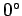C.

Table 2: Resistivities of some common metals atC.
 Material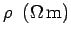Silver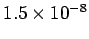Copper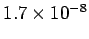Aluminium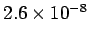Iron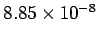Next: Emf and Internal Resistance Up: Electric Current Previous: Ohm's Law
Richard Fitzpatrick 2007-07-14